Select Page

### Quadratic Equations is an important topic in CAT Algebra. The best way to master a topic is to practice problems and we bring to you this set of 5 quadratic equation problems that you can use for the same.

Question 1: Let a, b, c be real numbers a≠0. If p is a root of a2y2+by + c = 0, q is the root of a2y2+by + c = 0 and 0 <p< q, then the equation a2y2+by + c = 0 has a root r that always satisfies

(a) r = (p+q)/2
(b) r = p + (q/2)
(c) r = a
(d) p <r <q

Explanation: (d)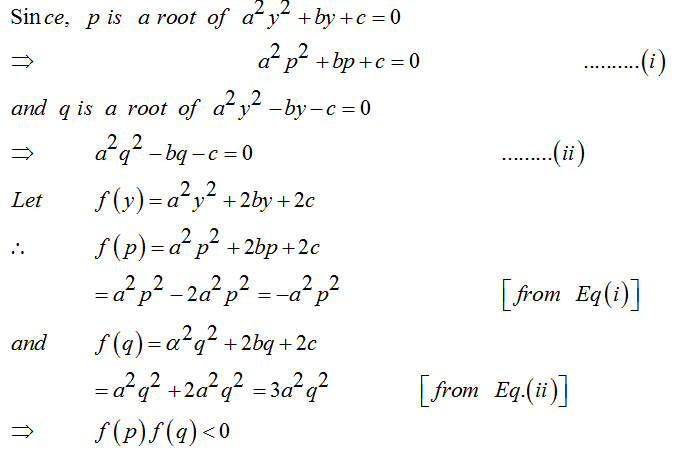ƒ(y) must have a root lying in the open interval (p, q).

Therefore p<r<q

Question 2: Let p, q be the roots of the equation (x-a)(x-b) = c, c is not equal to 0 . Then the roots of the equation (x-p)(x-q) + c = 0 are
(a) a, c
(b) b, c
(c) a, b
(d) a + c, b + c

Explanation: (c)

Given p, q are the roots of (x-a)(x-b) = c

(x-a)(x-b)-c = (x-p)(x-q)

(x-a)(x-b)= (x-p)(x-q)+c

a , b, are the roots of equation (x-p)(x-q)+c = 0

Question 3: If the roots of the equation x2-2bx+b2+b-3 = 0  are real and less than 3, then
(a) – 4 <b< 2
(b) 2<b< 3
(c) 0 <b< 4
(d) b> 3

Explanation: (a)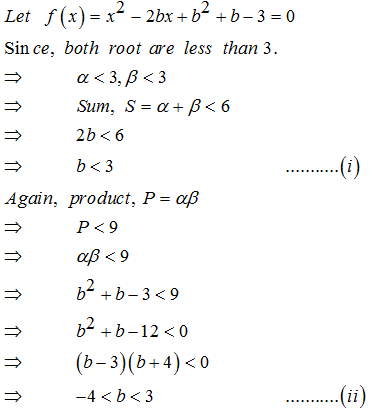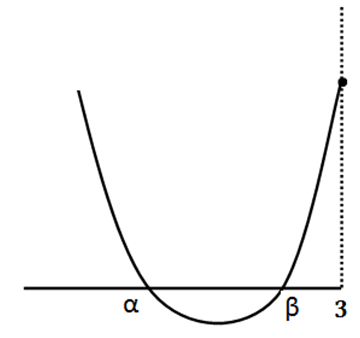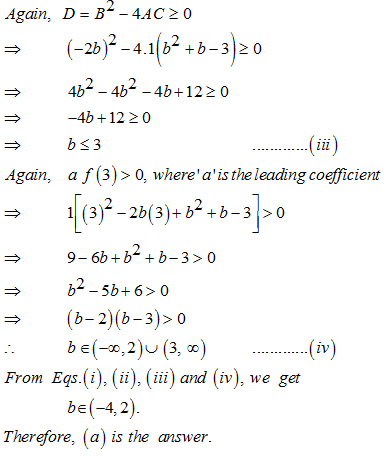Question 4:

If p and q (p<q) are the roots of the equation x2+bx+c = 0 ,  where c< 0 <b.
(a) 0<p<q
(b) p< 0 <q< |p|
(c) p < q< 0
(d) p < 0 |p| <q

Explanation: (b)

Given , c<0<b

Since ce, p+q = -b …………………………………..(1)

and pq = c

From equation 2, c<0

pq<0

=> Either p is –ve , q is +ve

Or, p is +ve , q is –ve .

From Eq. 1, b>0

=> -b <0

=> p+q <0 therfore the sum is negative

Now since sum is negative and one of p and q is negative and also p < q, we can conclude that p is negative and q is positive but |p| > q

Hence we have p< 0 <q< |p|

Question 5: For all ‘x’, x2+2px+(10 – 3p)>0, then the interval in which ‘p’ lies is
(a) p< –5
(b) –5 <p < 2
(c) p > 5
(d) 2 <p< 5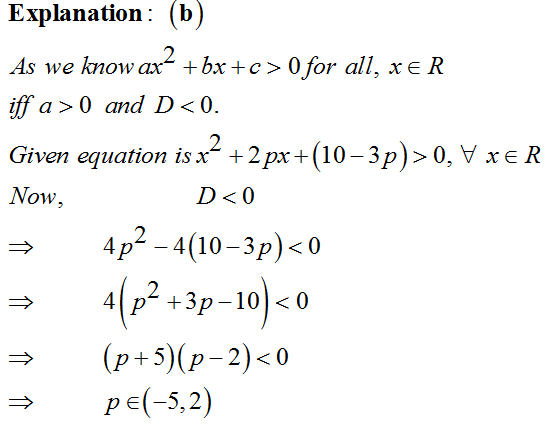Extra tips for CAT Quadratic Equations
• Quadratic equation is a top that massively features in class 11 and class 12 mathematics. In order to learn the absolute basics of the topic, it is recommended that you take up some basic level books from these classes.
• You can use class 11 NCERT or RD Sharma book to learn the basics of the topic.
• Make sure you also learn the graphical approach for these questions as this will help you master problems for this topic.

Get Posts Like This Sent to your Email
Updates for Free Live sessions and offers are sent on mail. Don't worry: we do not send too many emails..:)
Get Posts Like This Sent to your Email
Updates for Free Live sessions and offers are sent on mail. Don't worry: we do not send too many emails..:)